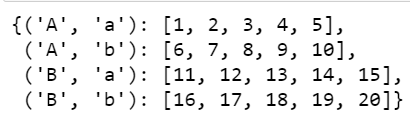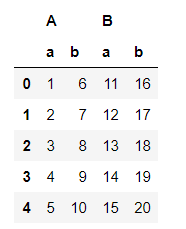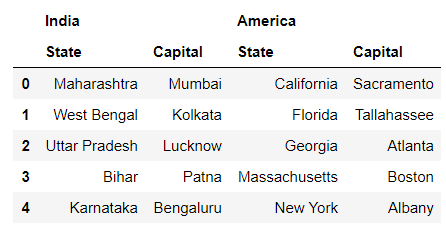Skip to content
Related Articles
Nested Dictionary to Multiindex Dataframe
• Last Updated : 24 Feb, 2021

Pandas DataFrame is a two-dimensional size-mutable, potentially heterogeneous tabular data structure with labeled axes (rows and columns). A Data frame is a two-dimensional data structure, i.e., data is aligned in a tabular fashion in rows and columns. Pandas DataFrame consists of three principal components, the data, rows, and columns. A Multiindex Dataframe is a pandas dataframe having multi-level indexing or hierarchical indexing.

Pandas needs multi-index values as tuples, not as a nested dictionary. So, first, we need to convert the nested index values into tuples.

Example #1:

## Python3

 `# Import module``import` `pandas as pd`` ` `# Nested dictionary to convert it``# into multiindex dataframe``nested_dict ``=` `{``'A'``: {``'a'``: [``1``, ``2``, ``3``,``                           ``4``, ``5``],``                     ``'b'``: [``6``, ``7``, ``8``,``                           ``9``, ``10``]},`` ` `               ``'B'``: {``'a'``: [``11``, ``12``, ``13``,``                           ``14``, ``15``],``                     ``'b'``: [``16``, ``17``, ``18``,``                           ``19``, ``20``]}}`` ` `reformed_dict ``=` `{}``for` `outerKey, innerDict ``in` `nested_dict.items():``    ``for` `innerKey, values ``in` `innerDict.items():``        ``reformed_dict[(outerKey,``                       ``innerKey)] ``=` `values`` ` `# Multiindex dataframe``reformed_dict`

Output:Notice that in the reformed_dict, index values are in the tuple. Now to convert reformed_dict into multiindex dataframe, we can use pd.DataFrame() method.

## Python3

 `multiIndex_df ``=` `pd.DataFrame(reformed_dict)``multiIndex_df`

Output:Here in the output, we can see the hierarchical index/ multi index for the column.

Example #2:

## Python3

 `# Import module``import` `pandas as pd`` ` `# Nested dictionary to convert it into multiindex dataframe``nested_dict ``=` `{``'India'``: {``'State'``: [``'Maharashtra'``, ``'West Bengal'``,``                                   ``'Uttar Pradesh'``, ``'Bihar'``, ``'Karnataka'``],``                         ``'Capital'``: [``'Mumbai'``, ``'Kolkata'``, ``'Lucknow'``,``                                     ``'Patna'``, ``'Bengaluru'``]},`` ` `               ``'America'``: {``'State'``: [``'California'``, ``'Florida'``, ``'Georgia'``,``                                     ``'Massachusetts'``, ``'New York'``],``                           ``'Capital'``: [``'Sacramento'``, ``'Tallahassee'``, ``'Atlanta'``,``                                       ``'Boston'``, ``'Albany'``]}}`` ` `reformed_dict ``=` `{}``for` `outerKey, innerDict ``in` `nested_dict.items():``    ``for` `innerKey, values ``in` `innerDict.items():``        ``reformed_dict[(outerKey, innerKey)] ``=` `values`` ` `# Display multiindex dataframe``multiIndex_df ``=` `pd.DataFrame(reformed_dict)``multiIndex_df`

Output:Attention geek! Strengthen your foundations with the Python Programming Foundation Course and learn the basics.

To begin with, your interview preparations Enhance your Data Structures concepts with the Python DS Course. And to begin with your Machine Learning Journey, join the Machine Learning – Basic Level Course

My Personal Notes arrow_drop_up